##### Trigonometry Workbook For DummiesYou can use the following six methods to prove that a quadrilateral is a rhombus. The last three methods in this list require that you first show (or be given) that the quadrilateral in question is a parallelogram:

• If all sides of a quadrilateral are congruent, then it’s a rhombus (reverse of the definition).

• If the diagonals of a quadrilateral bisect all the angles, then it’s a rhombus (converse of a property).

• If the diagonals of a quadrilateral are perpendicular bisectors of each other, then it’s a rhombus (converse of a property).

Tip: To visualize this one, take two pens or pencils of different lengths and make them cross each other at right angles and at their midpoints. Their four ends must form a diamond shape — a rhombus.

• If two consecutive sides of a parallelogram are congruent, then it’s a rhombus (neither the reverse of the definition nor the converse of a property).

• If either diagonal of a parallelogram bisects two angles, then it’s a rhombus (neither the reverse of the definition nor the converse of a property).

• If the diagonals of a parallelogram are perpendicular, then it’s a rhombus (neither the reverse of the definition nor the converse of a property).

Here’s a rhombus proof for you. Try to come up with a game plan before reading the two-column proof.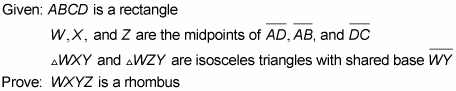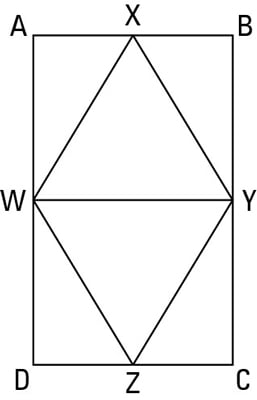Statement 1: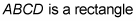Reason for statement 1: Given.

Statement 2: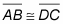Reason for statement 2: Opposite sides of a rectangle are congruent.

Statement 3: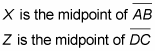Reason for statement 3: Given.

Statement 4: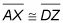Reason for statement 4: Like Divisions Theorem.

Statement 5: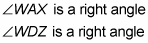Reason for statement 5: All angles of a rectangle are right angles.

Statement 6: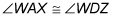Reason for statement 6: All right angles are congruent.

Statement 7: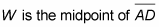Reason for statement 7: Given.

Statement 8: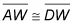Reason for statement 8: A midpoint divides a segment into two congruent segments.

Statement 9: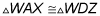Reason for statement 9: SAS, or Side-Angle-Side (4, 6, 8)

Statement 10: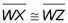Reason for statement 10: CPCTC (Corresponding Parts of Congruent Triangles are Congruent).

Statement 11: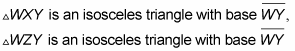Reason for statement 11: Given.

Statement 12: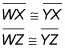Reason for statement 12: If a triangle is isosceles, then its two legs are congruent.

Statement 13: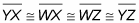Reason for statement 13: Transitivity (10 and 12).

Statement 14: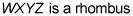Reason for statement 14: If a quadrilateral has four congruent sides, then it’s a rhombus.